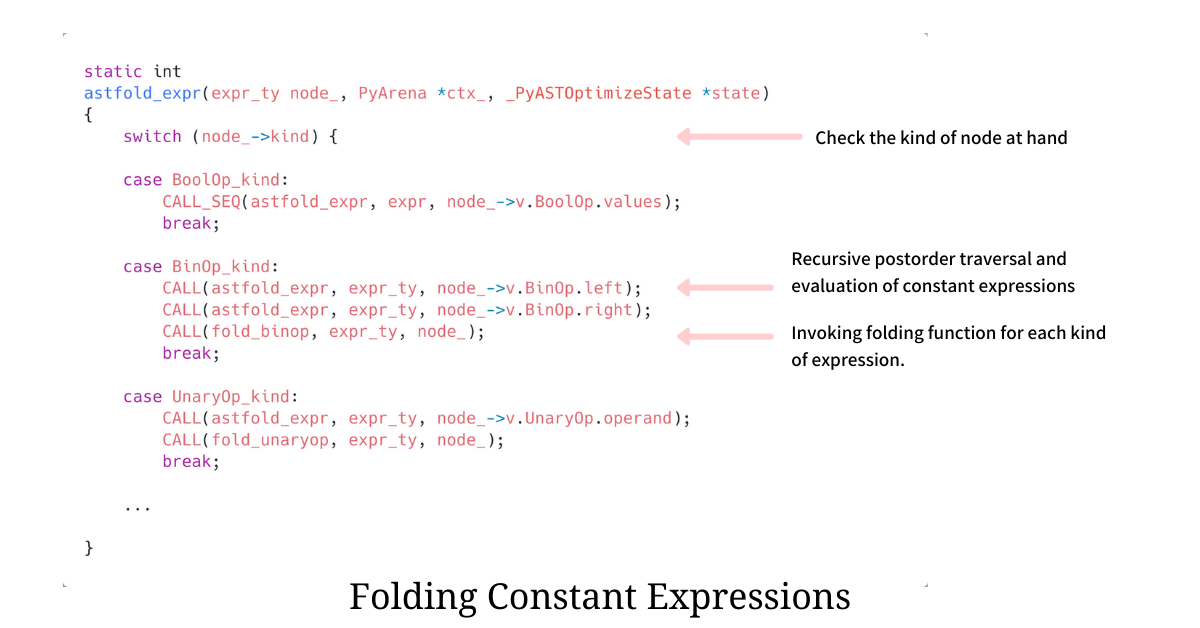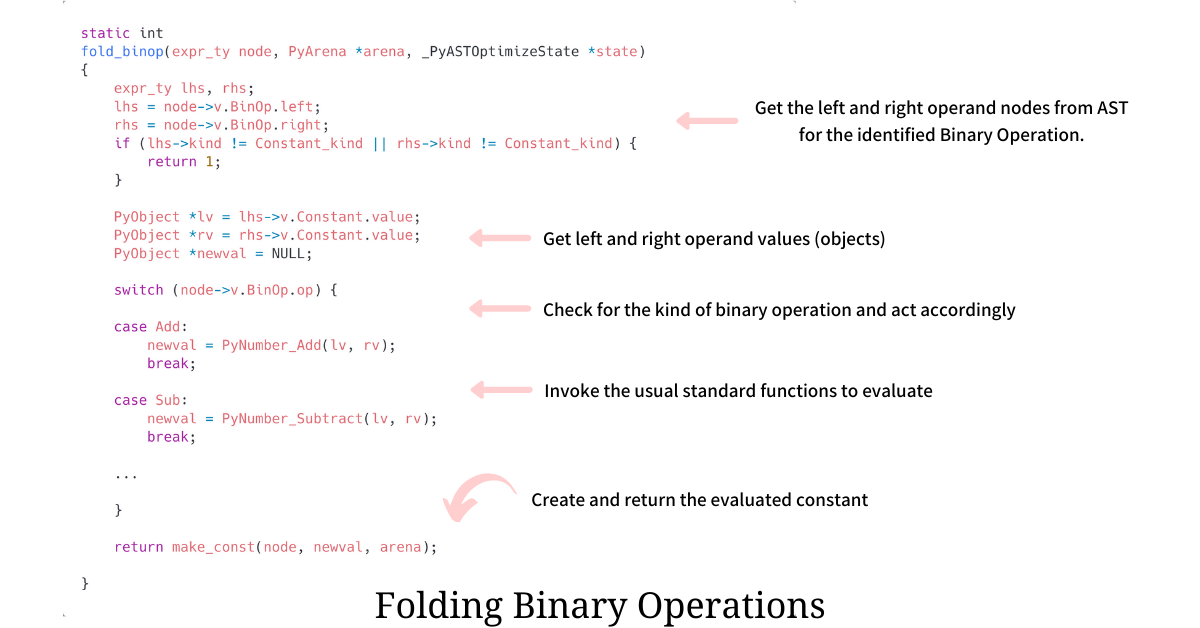Registrations for the November 2021 System Design Cohort are now open# Constant Folding in Python

Published on 10th Jan 2021

Every programming language aims to be performant in its niche and achieving superior performance requires a lot of compiler level optimizations. One famous optimization technique is Constant Folding where during compile time the engine tries to recognize constant expressions, evaluate them, and replaces the expression with this newly evaluated value, making the runtime leaner.

In this essay, we dive deep and find what exactly is Constant Folding, understand the scope of it in the world of Python and finally go through Python's source code - CPython - and find out how elegantly Python actually implements it.

# Constant Folding

In Constant Folding, the engine finds and evaluates constant expressions at compile time rather than computing them at runtime, making the runtime leaner and faster.

``````>>> day_sec = 24 * 60 * 60
``````

When the compiler encounters a constant expression, like above, it evaluates the expression and replaces it with the evaluated value. The expression is usually replaced by the evaluated value in the Abstract Syntax Tree, but the implementation is totally up to the language. Hence the above expression is effectively executed as

``````>>> day_sec = 86400
``````

# Constant Folding in Python

In Python, we could use the Disassembler module to get the CPython bytecode giving us a good peek at how things will be executed. When we disassemble the above constant expression using the `dis` module, we get the following bytecode

``````>>> import dis
>>> dis.dis("day_sec = 24 * 60 * 60")

2 STORE_NAME               0 (day_sec)
6 RETURN_VALUE
``````

We see that the bytecode, instead of having two binary multiply operations followed by one `LOAD_CONST`, is having just one `LOAD_CONST` with the already evaluated value of `86400`. This indicates that the CPython interpreter during parsing and building of Abstract Syntax Tree folded the constant expression, `24 * 60 * 60` and replaced it with the evaluated value `86400`.

### Scope of Constant Folding

Python tries to fold every single constant expression present but there are some cases where even though the expression is constant, but Python chooses not to fold it. For example, Python does not fold `x = 4 ** 64` while it does fold `x = 2 ** 64`.

Apart from the arithmetic expressions, Python also folds expressions involving Strings and Tuples, where constant string expressions till the length `4096` are folded.

``````>>> a = "-" * 4096   # folded
>>> a = "-" * 4097   # not folded
>>> a = "--" * 4096  # not folded
``````

# Internals of Constant Folding

Now we shift our focus to the internals and find exactly where and how CPython implements Constant Folding. All AST optimizations, including Constant Folding, can be found in file ast_opt.c. The base function starting it all is `astfold_expr` which folds any and every expression that Python source has. The function recursively goes through the AST and tries to fold every constant expression, as seen in the snippet below.The `astfold_expr` before folding the expression at hand, tries to fold its child expressions (operands) and then delegates the folding to the corresponding specific expression folding function. The operation-specific folding function evaluates the expression and returns the evaluated constant value, which is then put into the AST.

For example, whenever `astfold_expr` encounters a binary operation, it recursively folds the two child operands (expressions) before evaluating the expression at hand using `fold_binop`. The function `fold_binop` returns the evaluated constant value as seen in the snippet below.`fold_binop` function folds the binary operation by checking the kind of operator at hand and then invoking the corresponding evaluation function on them. For example, if the operation at hand is an addition then, to evaluate the final value, it invokes `PyNumber_Add` on both its left and right operands.

### What makes this elegant?

Instead of writing special logic to handle certain patterns or types to fold constant expressions efficiently, CPython invokes the same general code. For example, it invokes the same usual `PyNumber_Add` function while folding that it does to perform the usual addition operation.

CPython has thus eradicated the need to write special functions to handle constant folding by making sure its code and evaluation process is structured in such a way that the general-purpose code itself can handle the evaluation of constant expressions.

# References

If my work adds value, consider supporting me## 2000+ Subscribers

If you like what you read subscribe you can always subscribe to my newsletter and get the post delivered straight to your inbox. I write essays on various engineering topics and share it through my weekly newsletter 👇

# Other articles that you might likePersonalization is what we all love. In this article we find how we could personalize the Python int...

21st FebInteger Caching in Python

To gain a performance boost and avoid reallocation of frequently used integers, Python creates singl...

17th MayMaking Python Integers Iterable

In Python, Integers are not iterables but we can make them iterable by implementing __iter__ functio...

14th JunBuilding Finite State Machines with Python Coroutines

The most intuitive way of building and implementing Finite State Machines is by using Python Corouti...

19th Apr